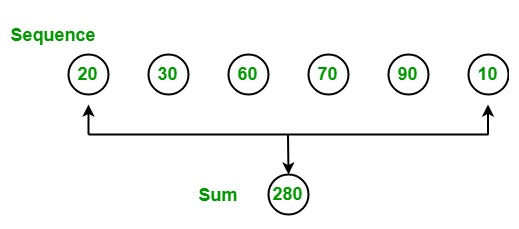Open in App
Not now

# Aggregation Function in LINQ

• Last Updated : 26 May, 2019

In LINQ, aggregation functions are those functions which are used to calculate a single value from the collection of the values. Real life example of aggregation function is calculating the annual rainfall occurred in 2018 according to readings collected whole year. Another example, the sum function is used to find the sum of the values present in the given array or sequence.Following is the list of the methods that are used to perform aggregation operations:

Method Description
Aggregate It performs, a custom aggregation operation on the values of a collection.
Average It calculates the average value of a collection of values.
Count It counts the elements in a collection, optionally only those elements that satisfy a predicate function.
LongCount It counts the elements in a large collection, optionally only those elements that satisfy a predicate function.
Max It determines the maximum value in a collection.
Min It determines the minimum value in a collection.
Sum It calculates the sum of the values in a collection.

Example 1:

 `// C# program to illustrate how to ` `// find the sum of the given sequence ` `using` `System; ` `using` `System.Linq; ` ` `  `public` `class` `GFG { ` ` `  `    ``// Main Method ` `    ``static` `public` `void` `Main() ` `    ``{ ` ` `  `        ``int``[] sequence = {20, 40, 50, 68, 90,  ` `                          ``89, 99, 9, 57, 69}; ` ` `  `        ``Console.WriteLine(``"The sum of the given sequence is: "``); ` ` `  `        ``// Finding sum of the given sequence ` `        ``// Using Sum function ` `        ``int` `result = sequence.Sum(); ` `        ``Console.WriteLine(result); ` `    ``} ` `} `

Output:

```The sum of the given sequence is:
591
```

Example 2:

 `// C# program to illustrate how to  ` `// find the minimum and maximum value ` `// from the given sequence ` `using` `System; ` `using` `System.Linq; ` ` `  `public` `class` `GFG { ` ` `  `    ``// Main Method ` `    ``static` `public` `void` `Main() ` `    ``{ ` ` `  `        ``int``[] sequence = {201, 39, 50, 9, 7, 99}; ` ` `  `        ``// Display the Sequence ` `        ``Console.WriteLine(``"Sequence is: "``); ` ` `  `        ``foreach``(``int` `s ``in` `sequence) ` `        ``{ ` `            ``Console.WriteLine(s); ` `        ``} ` ` `  `        ``// Finding the minimum value ` `        ``// from the given sequence ` `        ``// Using Min function ` `        ``int` `result1 = sequence.Min(); ` ` `  `        ``Console.WriteLine(``"Minimum Value is: {0}"``, result1); ` ` `  `        ``// Finding the maximum value  ` `        ``// from the given sequence ` `        ``// Using Max function ` `        ``int` `result2 = sequence.Max(); ` ` `  `        ``Console.WriteLine(``"Maximum Value is: {0}"``, result2); ` `    ``} ` `} `

Output:

```Sequence is:
201
39
50
9
7
99
Minimum Value is: 7
Maximum Value is: 201
```

Reference:

My Personal Notes arrow_drop_up
Related Articles